© | << < ? > >> | Dror Bar-Natan: Talks:

## Quantum Probability

### University of Toronto, October 2, 2003

We start by loading a necessary Mathematica package, by defininig the tensor product `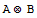` of two matrices A and B and the 2×2 identity matrix  ``:

In:=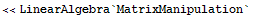In:=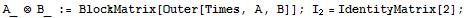Next we define the unit "probability vector" `v,` and our observables ("random variables")and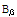as tensor products of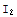with some prescribed:

In:=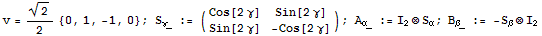In:=Out=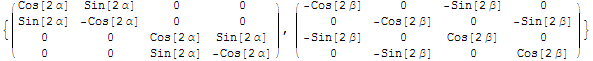We check that both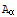andare (±1)-valued and have zero mean, hence both attain +1 and -1 with 50-50 chance:

In:=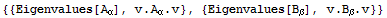Out=The's and the's commute, hence they have a joint distribution! Indeed,

In:=Out=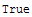The's and the's are both (±1)-valued, so the probability that they are equal is the expectation value (mean) of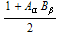:

In:=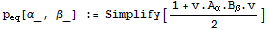Finally, the following is stricktly impossible, classically speaking:

In:=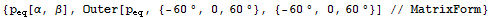Out=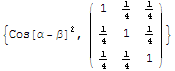See also N. D. Mermin,  Physics Today 39(4) 38 (1985) and D. Bar-Natan, Foundations of Physics 19(1) 97 (1989).

Converted by Mathematica  (October 1, 2003)